# The Straight Line Solutions Exercise 3(c) Inter

The Straight Line Exercise 3(c) Maths 1b textbook Chapter 3 Inter Solutions are given.

Study the textbook lesson The Straight Line very well.

All problems with answers are given. These are very simple to understand.

Observe the example problems and solutions in the textbook. Try them well.

Observe the given solutions and try them in your own method.

Maths 1b Inter solutions

Locus

Exercise 1(a)

Transformations of axes

Exercise 2(a)

The Straight Line

Exercise 3(a)

Three Dimensional Coordinates

Exercise 5(a)

Exercise 5(b)

Direction Cosines and Direction Ratios

Exercise 6(a)

Exercise 6(b)

The Plane

Exercise 7(a)

Limits and Continuity

Exercise 8(a)

Exercise 8(b)

Exercise 8(c)

Exercise 8(d)

Exercise 8(e)

M

You can see the solutions for text book Maths 1A

Functions

Exercise 1(a)

Exercise 1(b)

Exercise 1(c)

Mathematical Induction

Exercise 2(a)

Matrices

Exercise 3(a)

Exercise 3(b)

Exercise 3(c)

Exercise 3(d)

Exercise 3(e)

Exercise 3(f)

Exercise 3(g)

Exercise 3(h)

Exercise 3(i)

Exercise 4(a)

Exercise 4(b)

Product of Vectors

Exercise 5(a)

Exercise 5(b)

Exercise 5(c)

Trigonometric Ratios up to Transformations

Exercise 6(a)

Exercise 6(b)

Exercise 6(c)

Exercise 6(d)

Exercise 6(e)

Exercise 6(f)

Trigonometric Equations

Exercise 7(a)

Inverse Trigonometric Equations

Exercise 8(a)

Hyperbolic Functions

Exercise 9(a)

Properties of Triangles

Exercise 10(a)

Exercise 10(b)

SSC Maths text book Solutions class 10

Inter Maths 1A solutions

Inter Maths 1A textbook solutions

Inter Maths 1B solutions

Inter Maths 1IA text book solutions

Inter Maths IIB text book solutions

M

# Exercise 3(c) The Straight Line Solutions Inter

Chapter 3 The Straight Line

Exercise 3(c)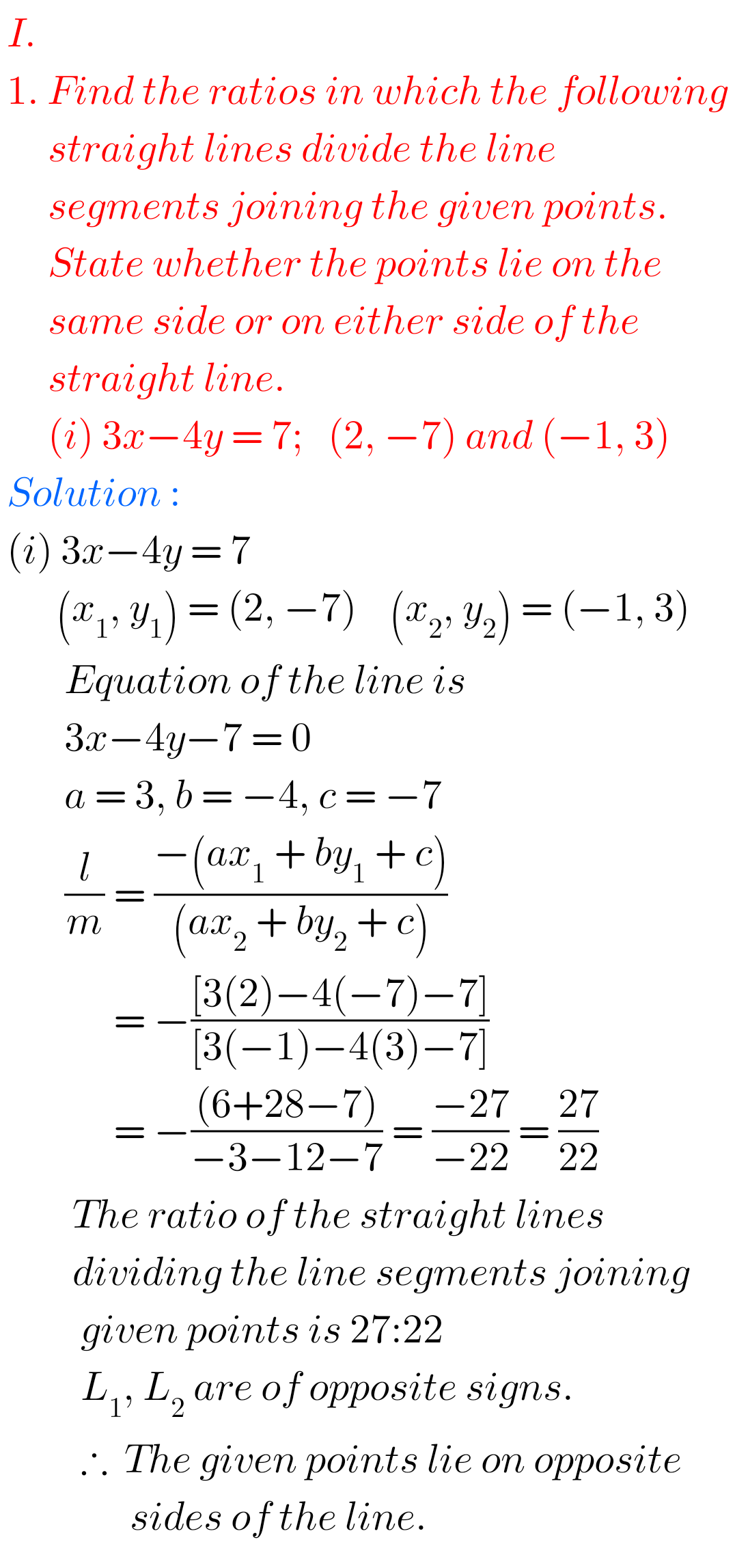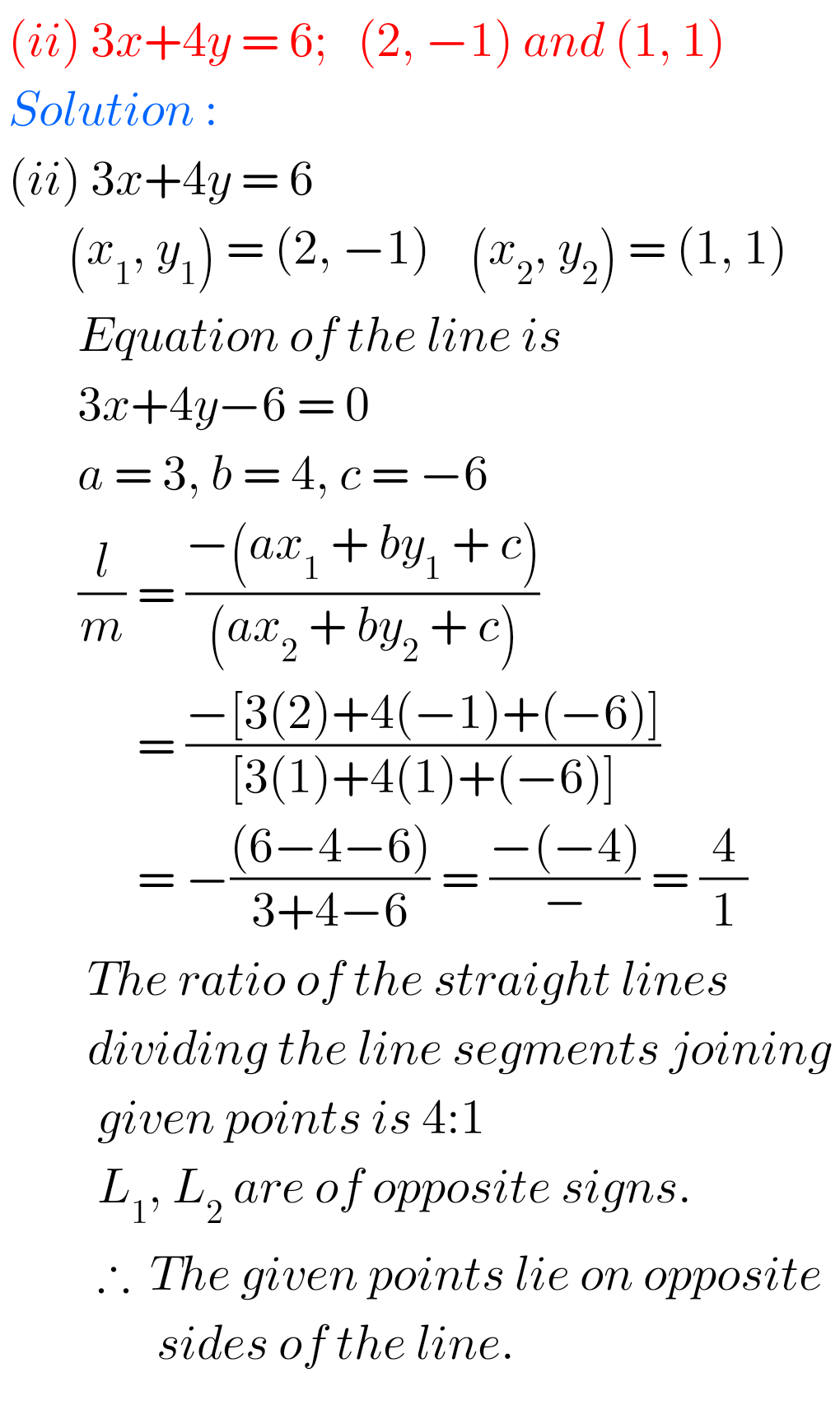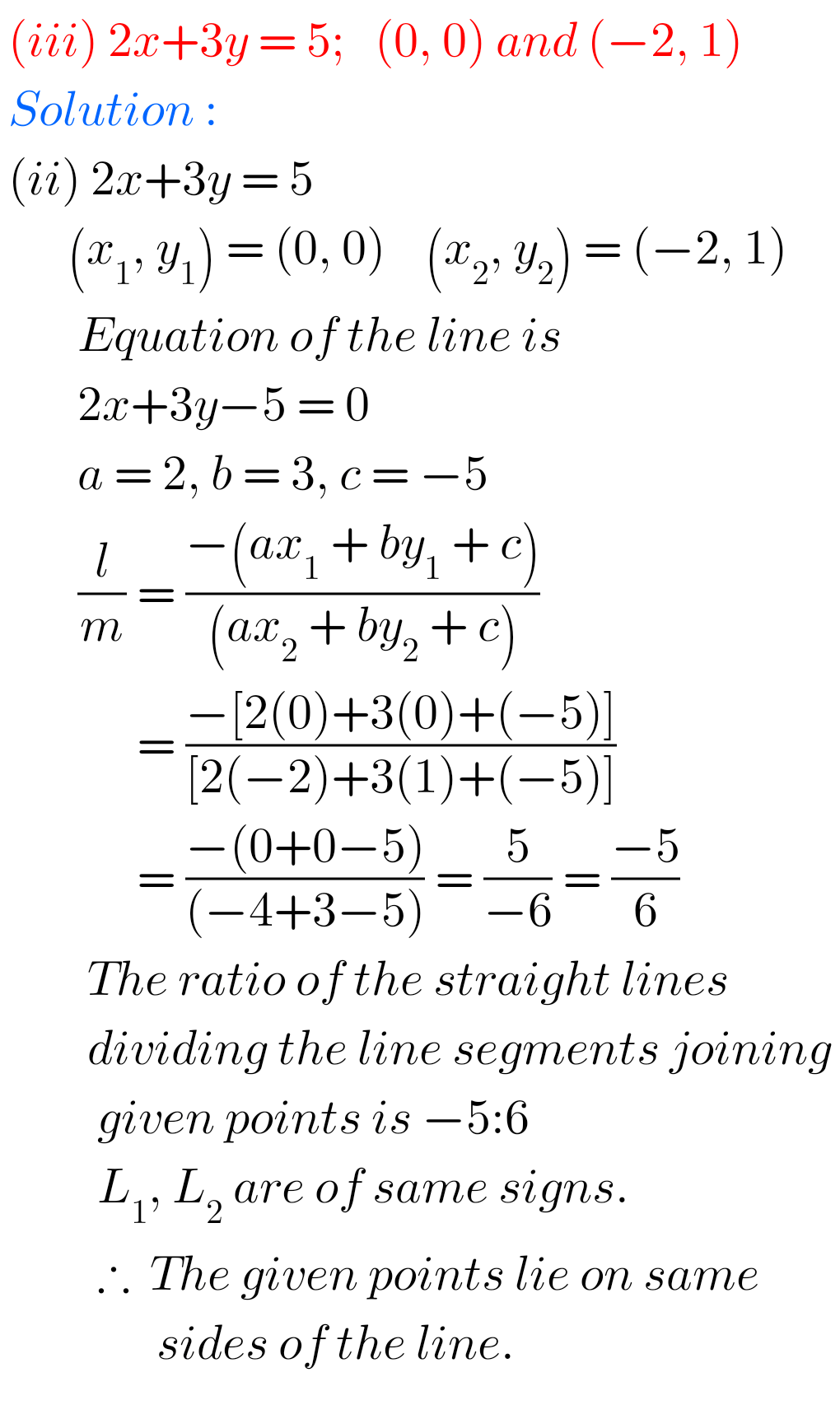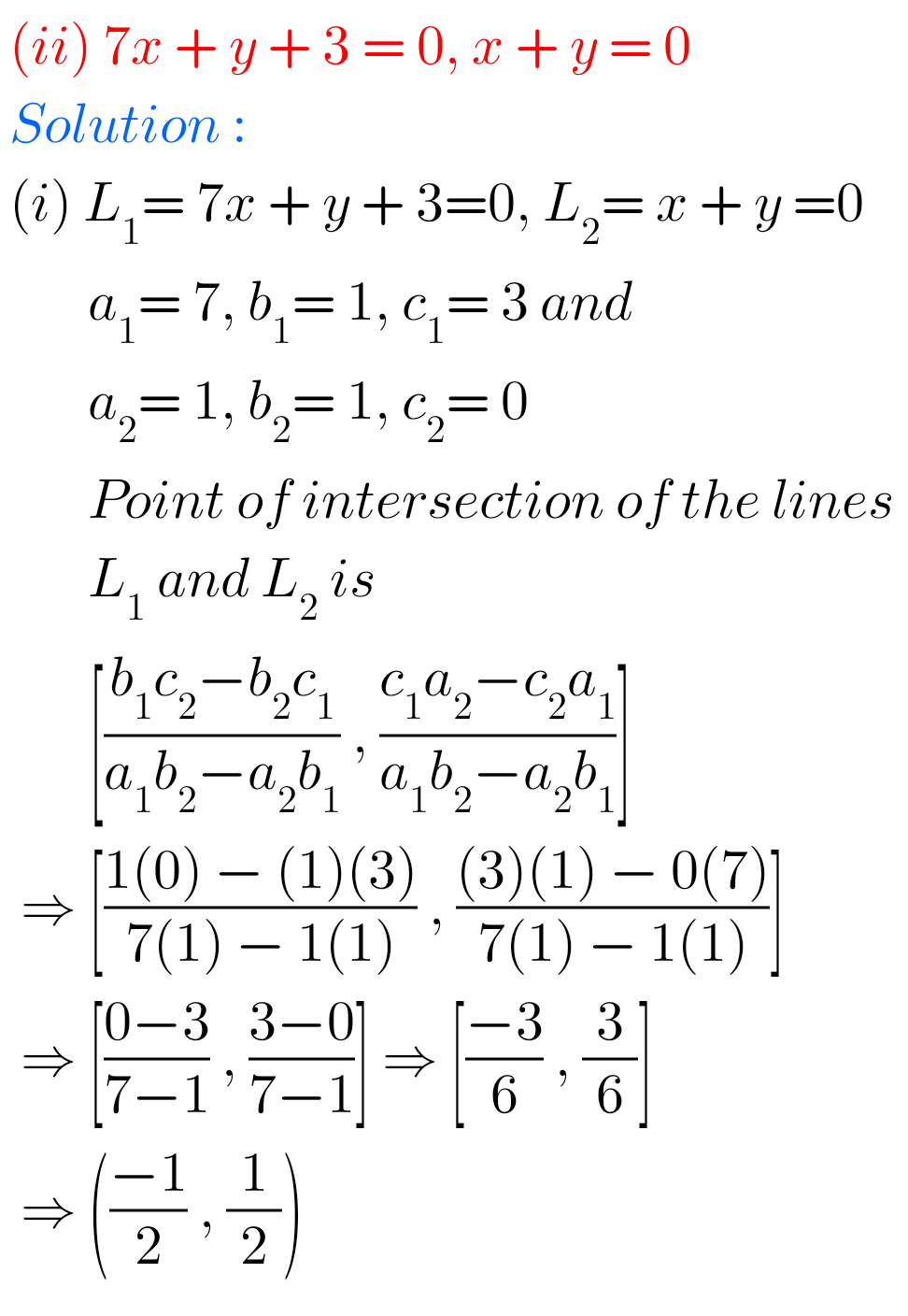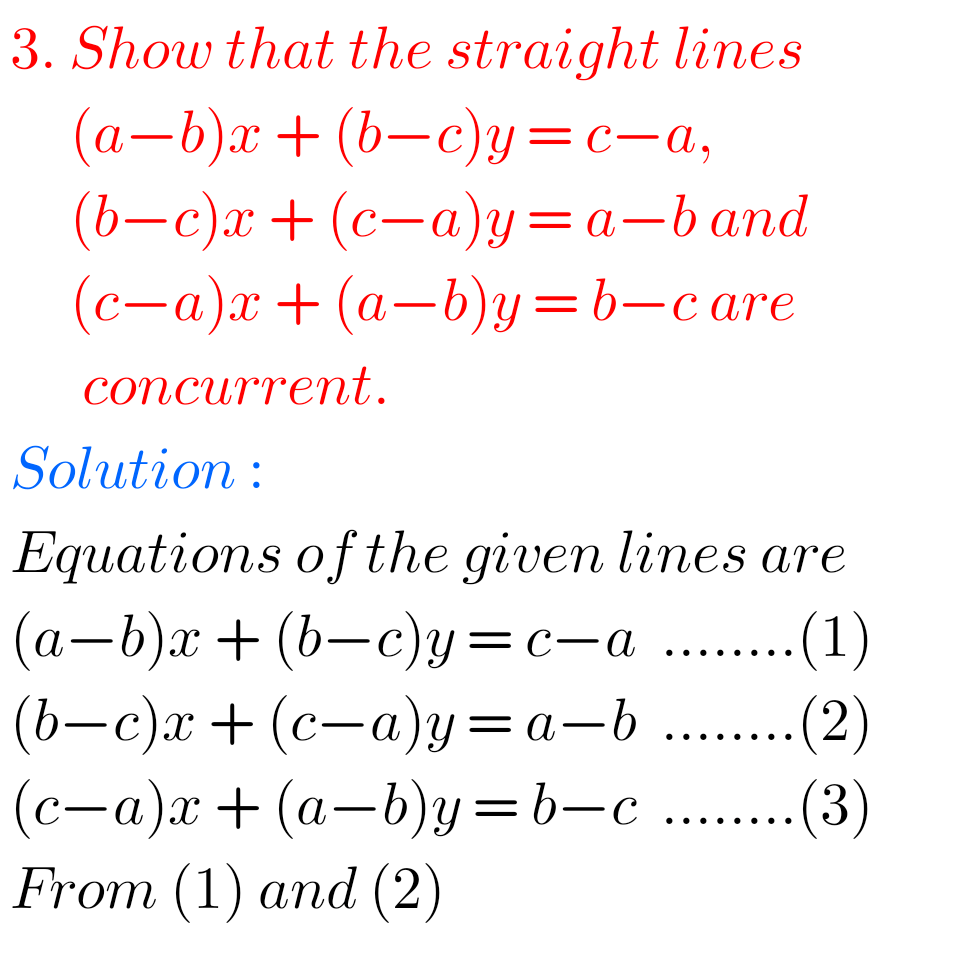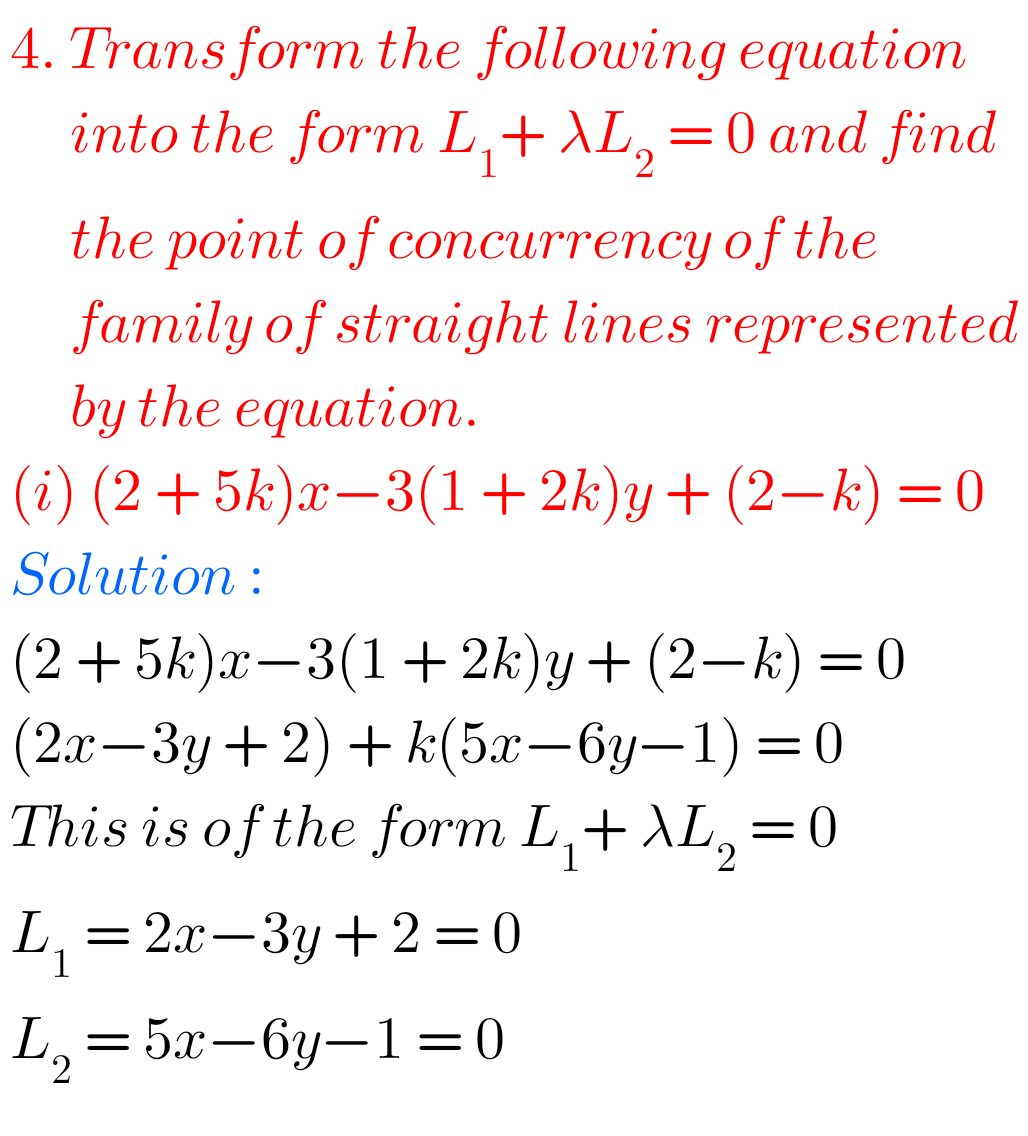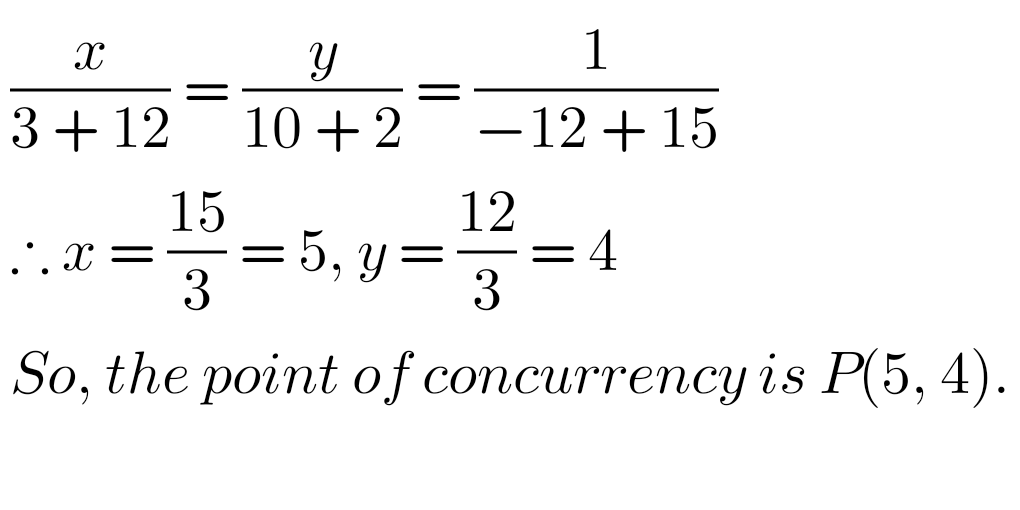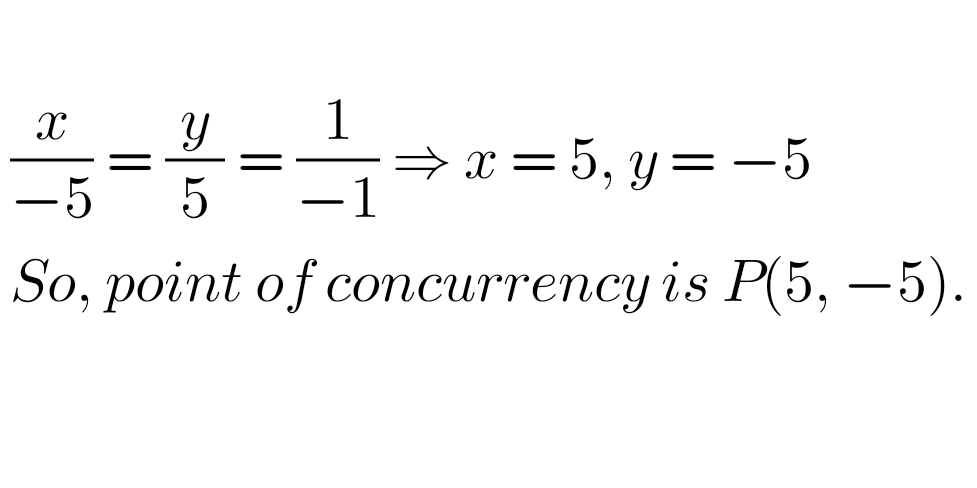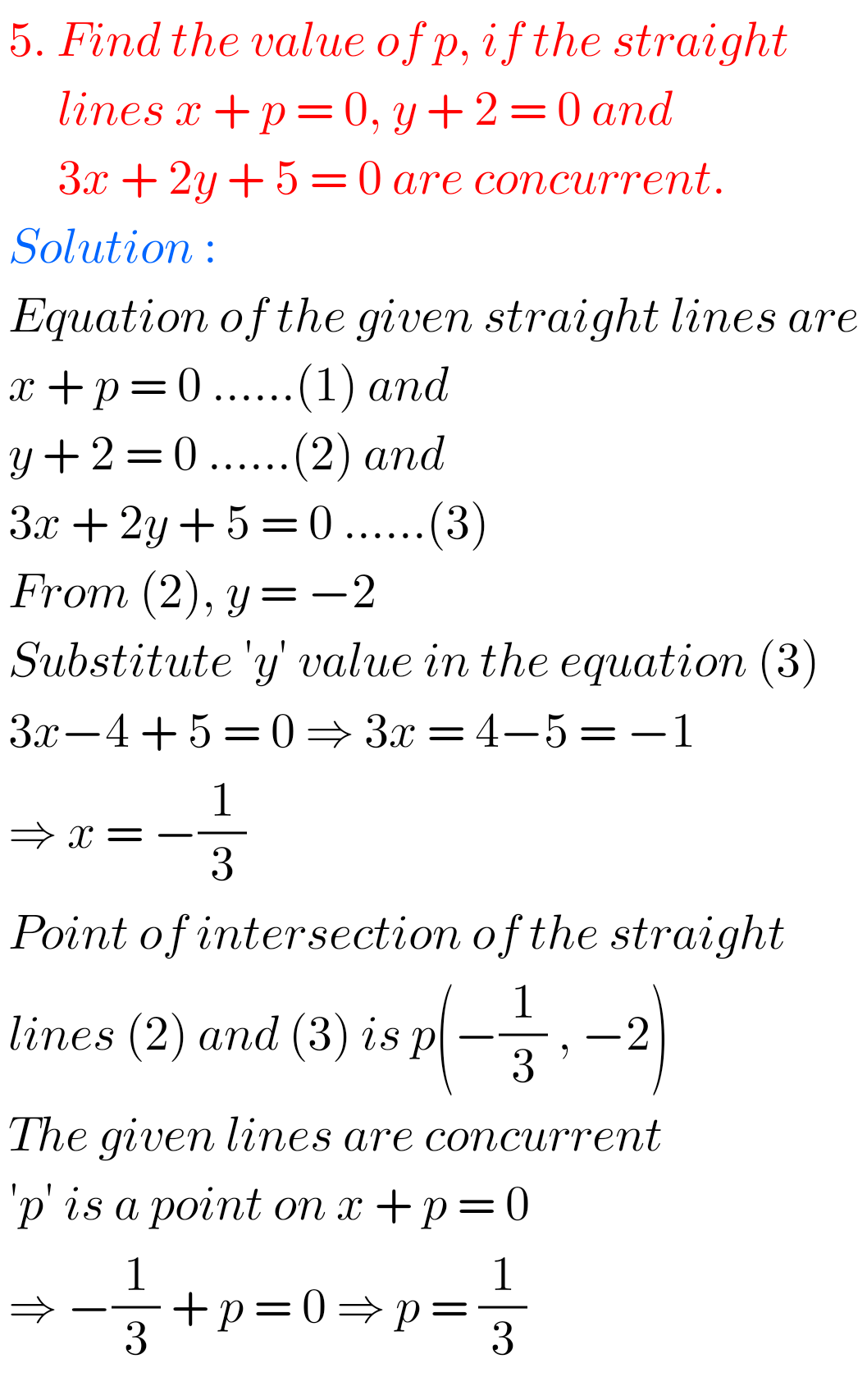## Solutions for The Straight Line Inter Maths 1b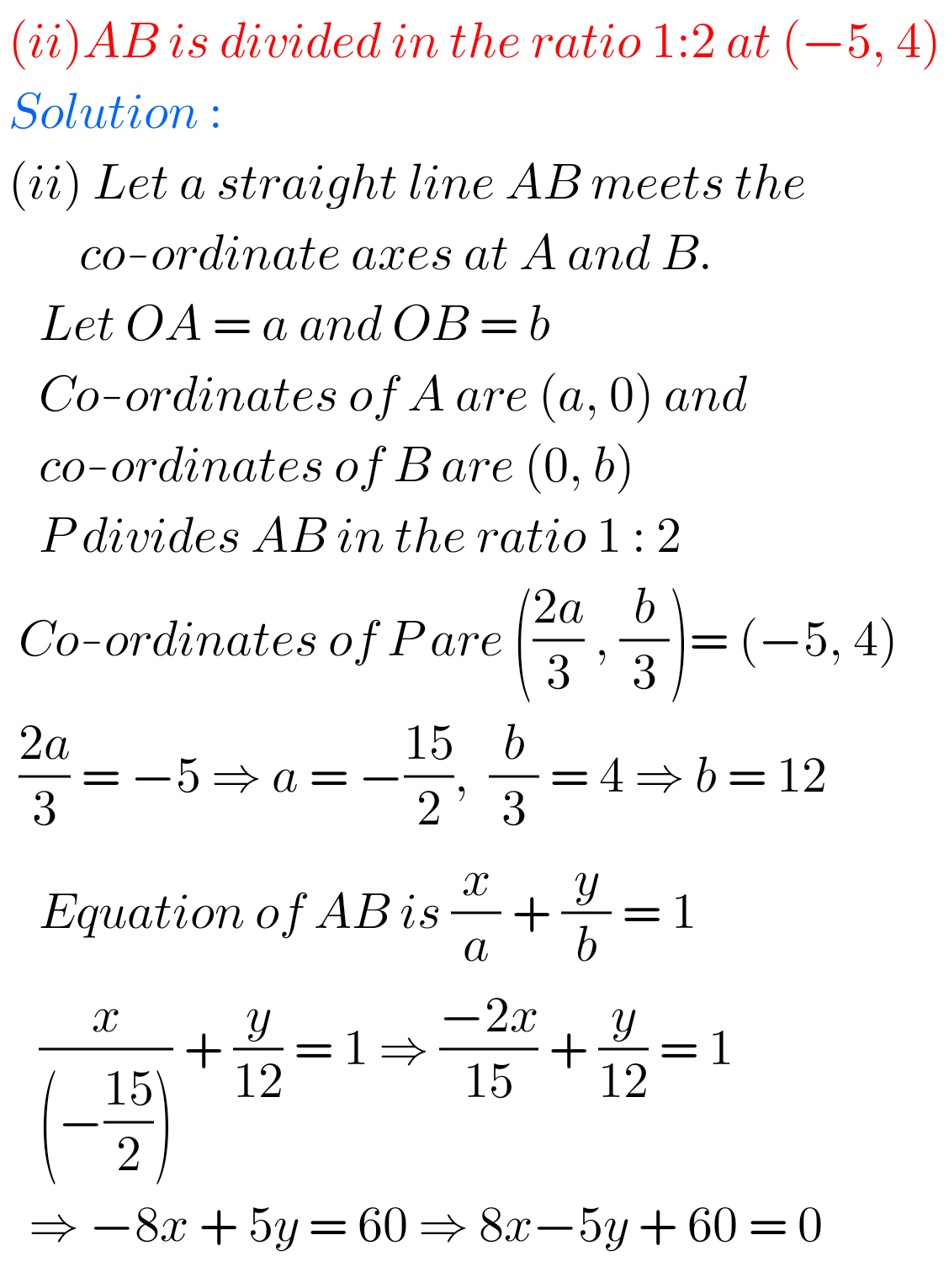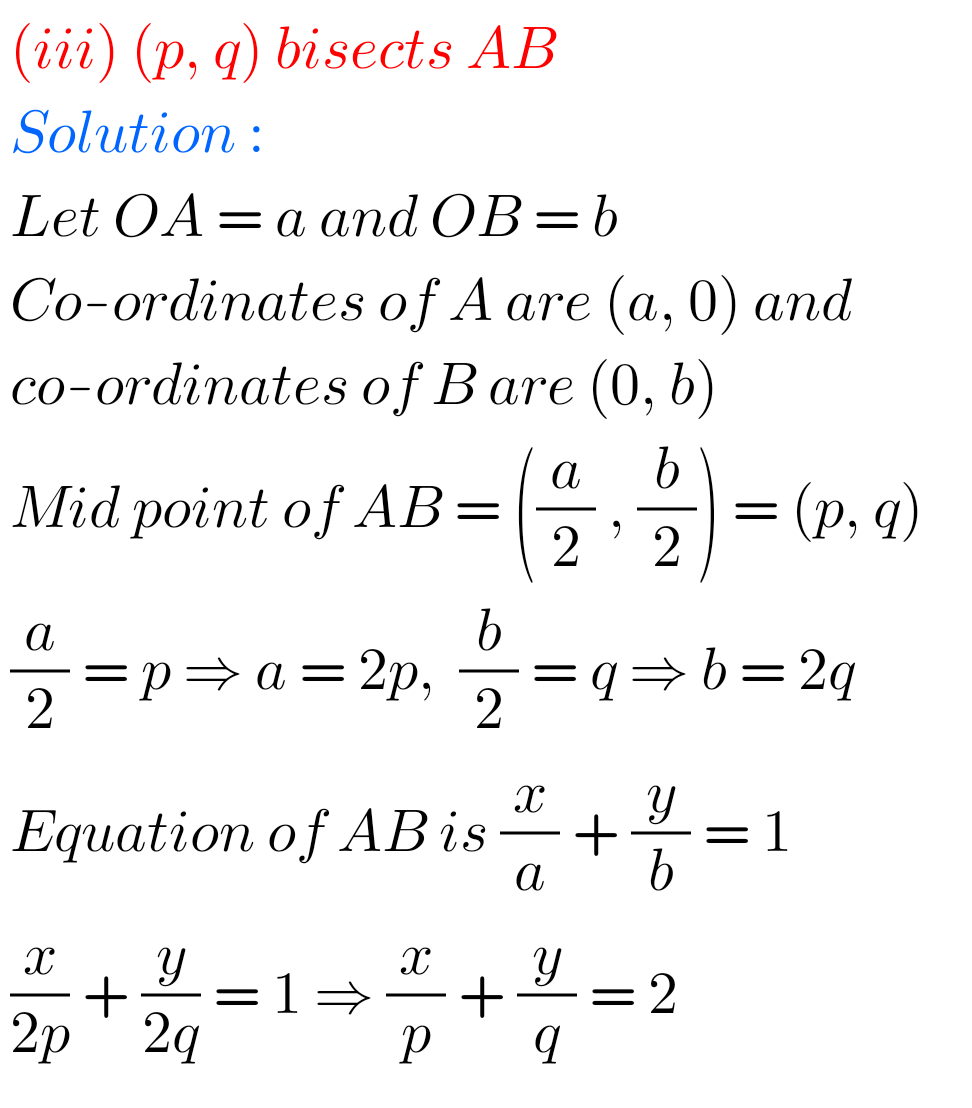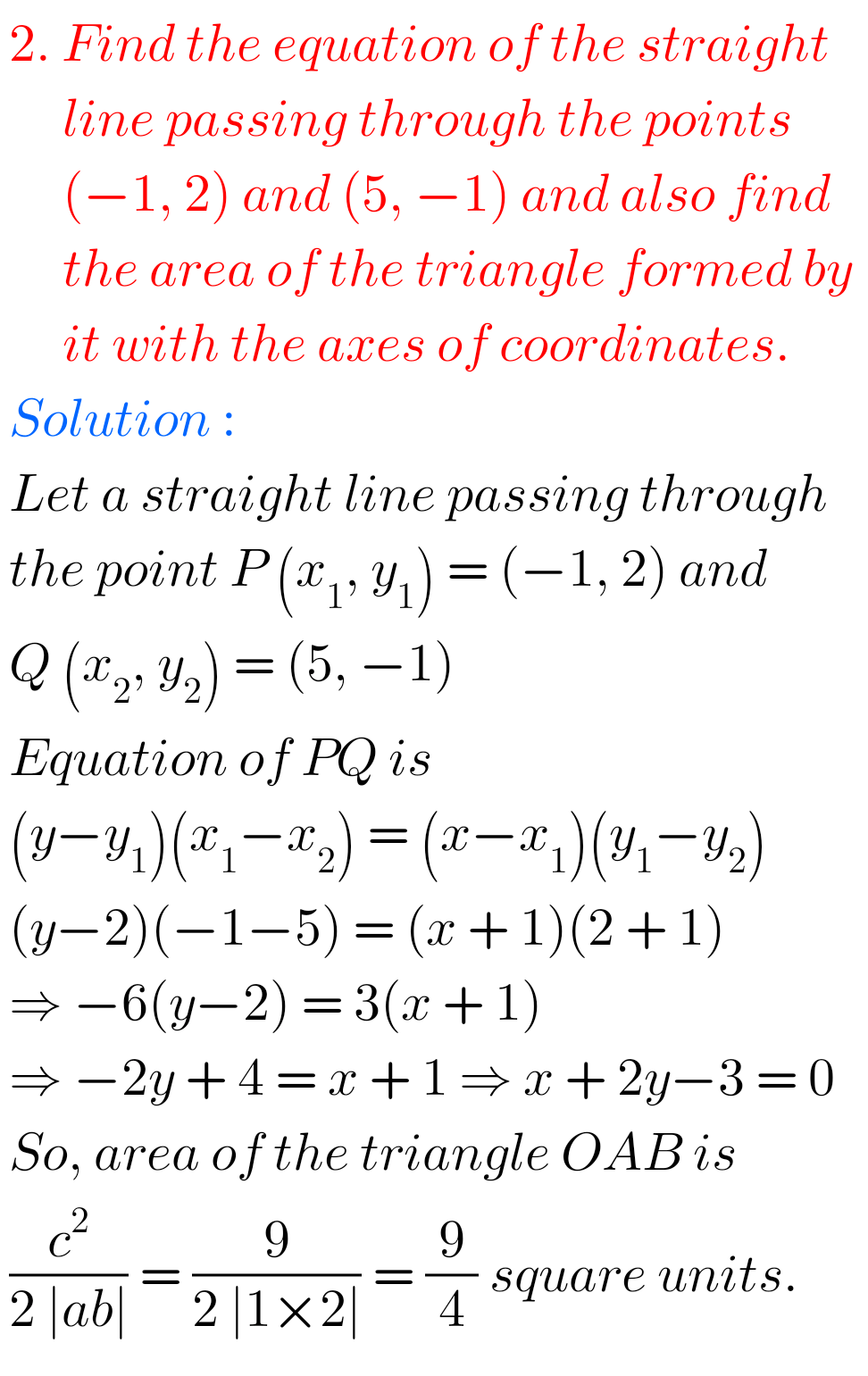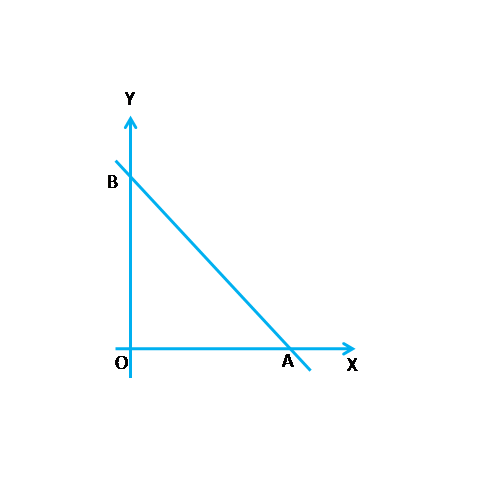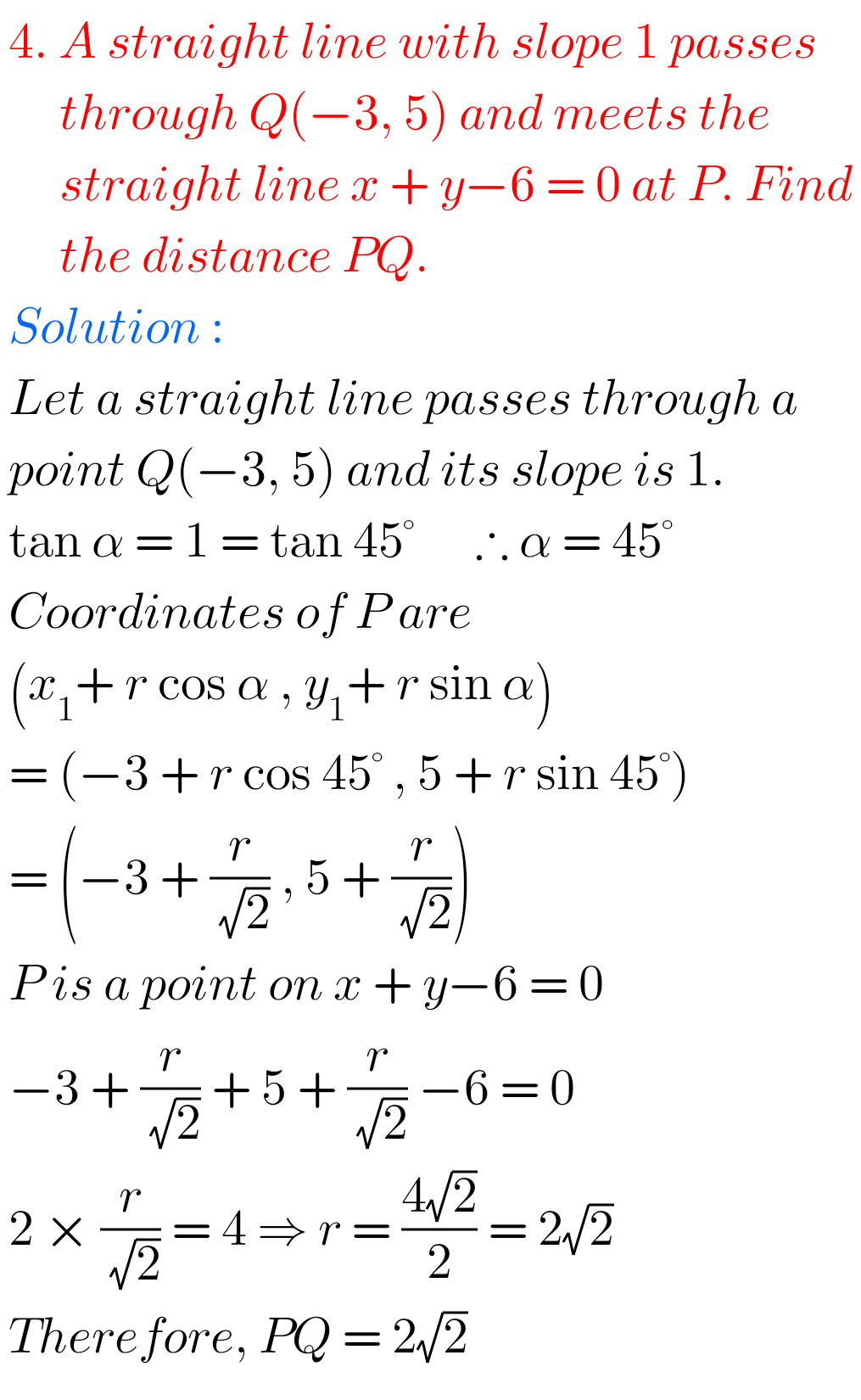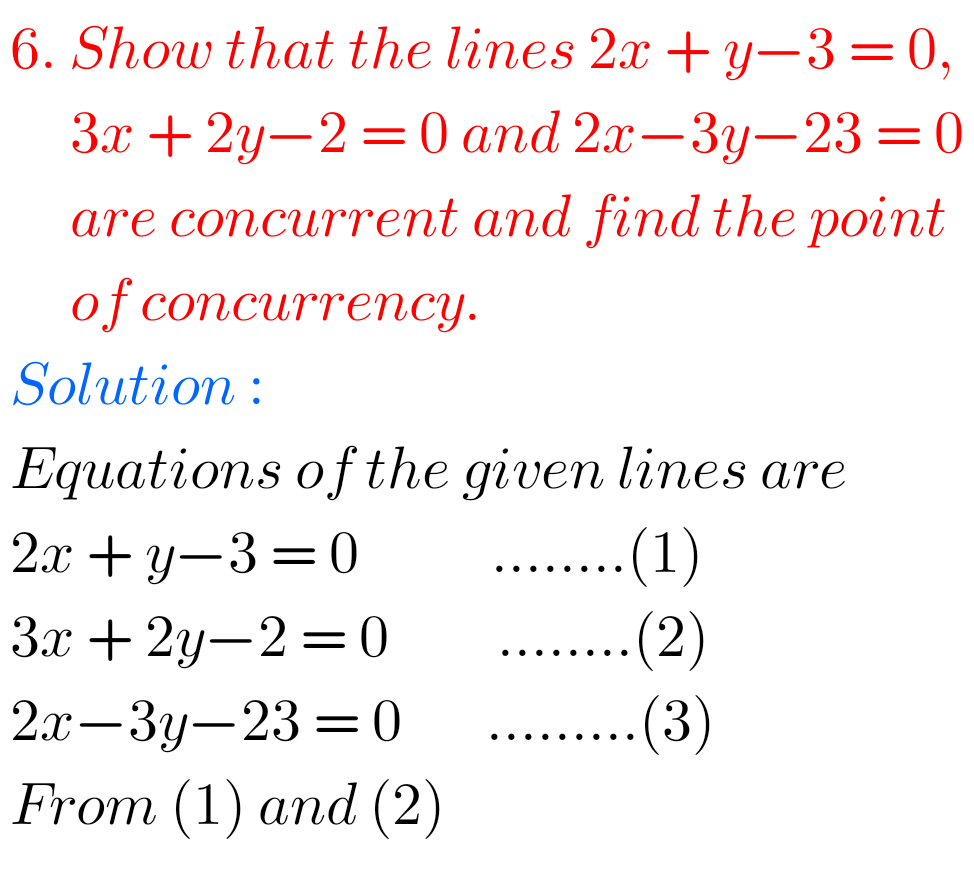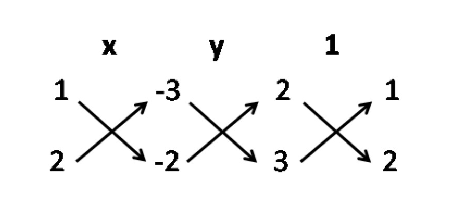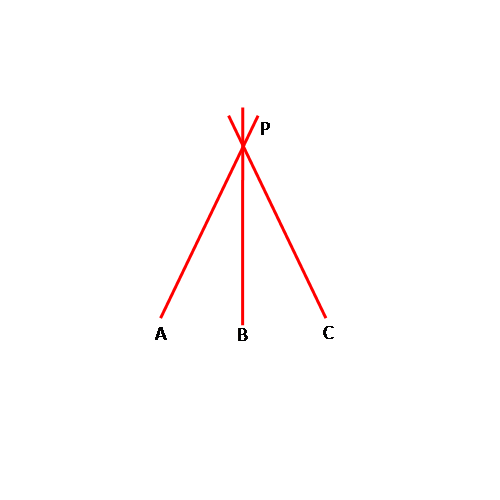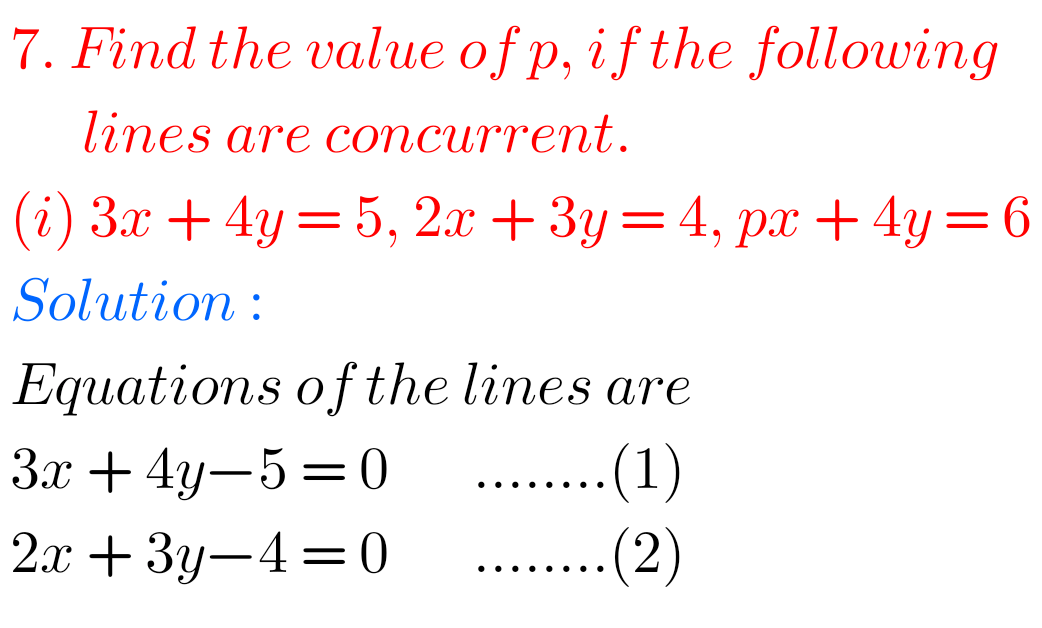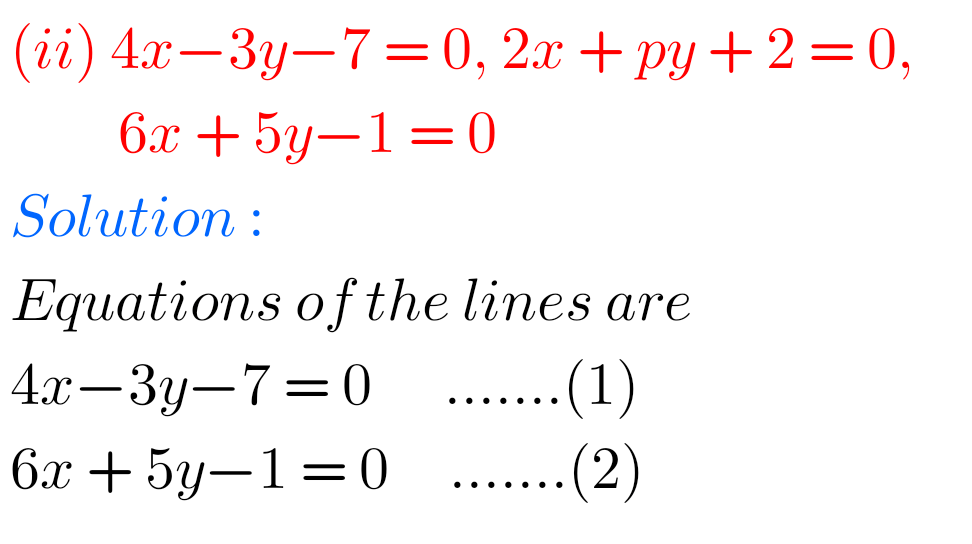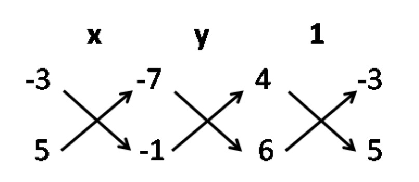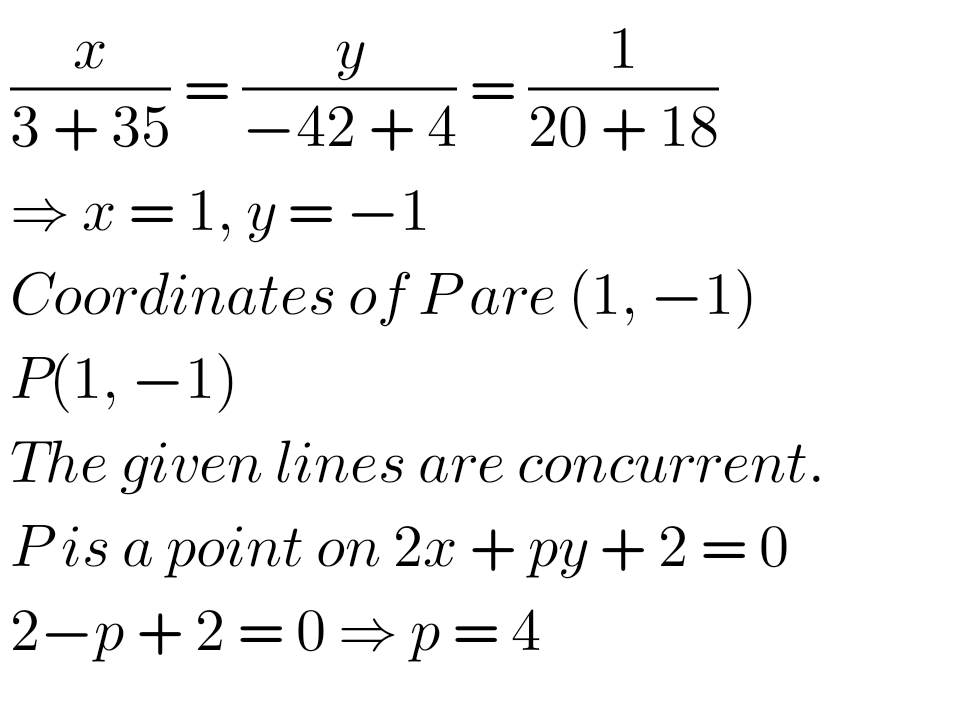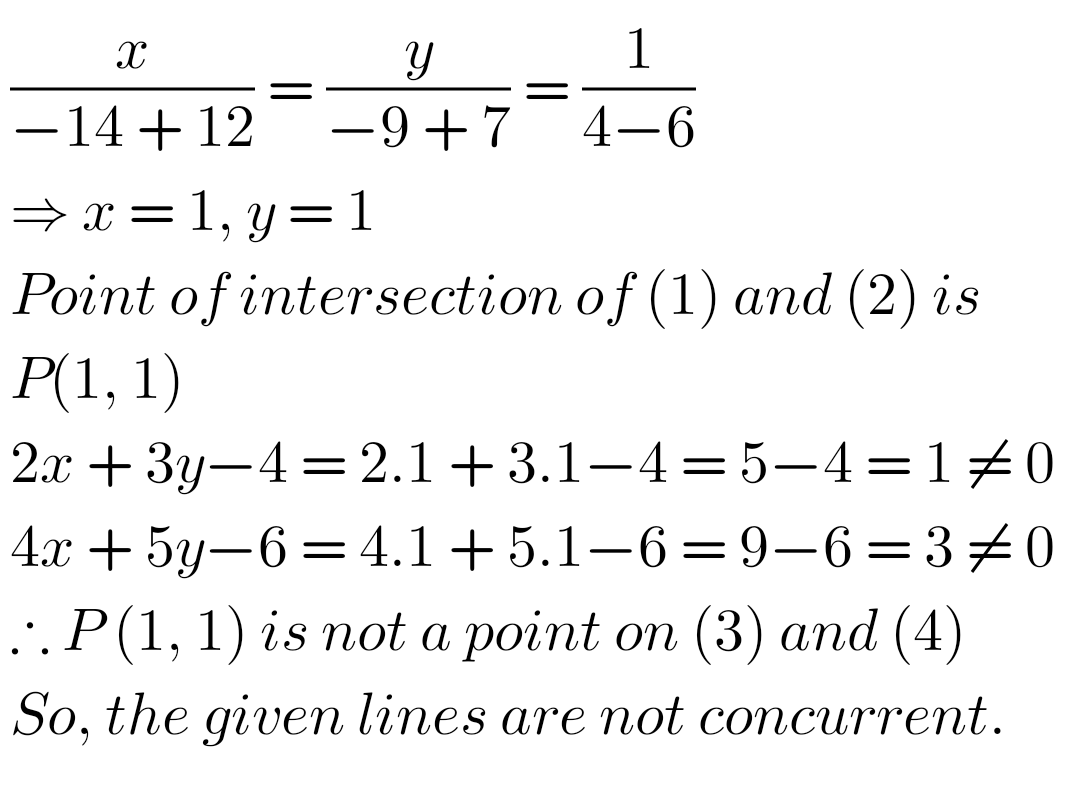## Intermediate Maths Solutions for The Straight Line Exercise 3(c)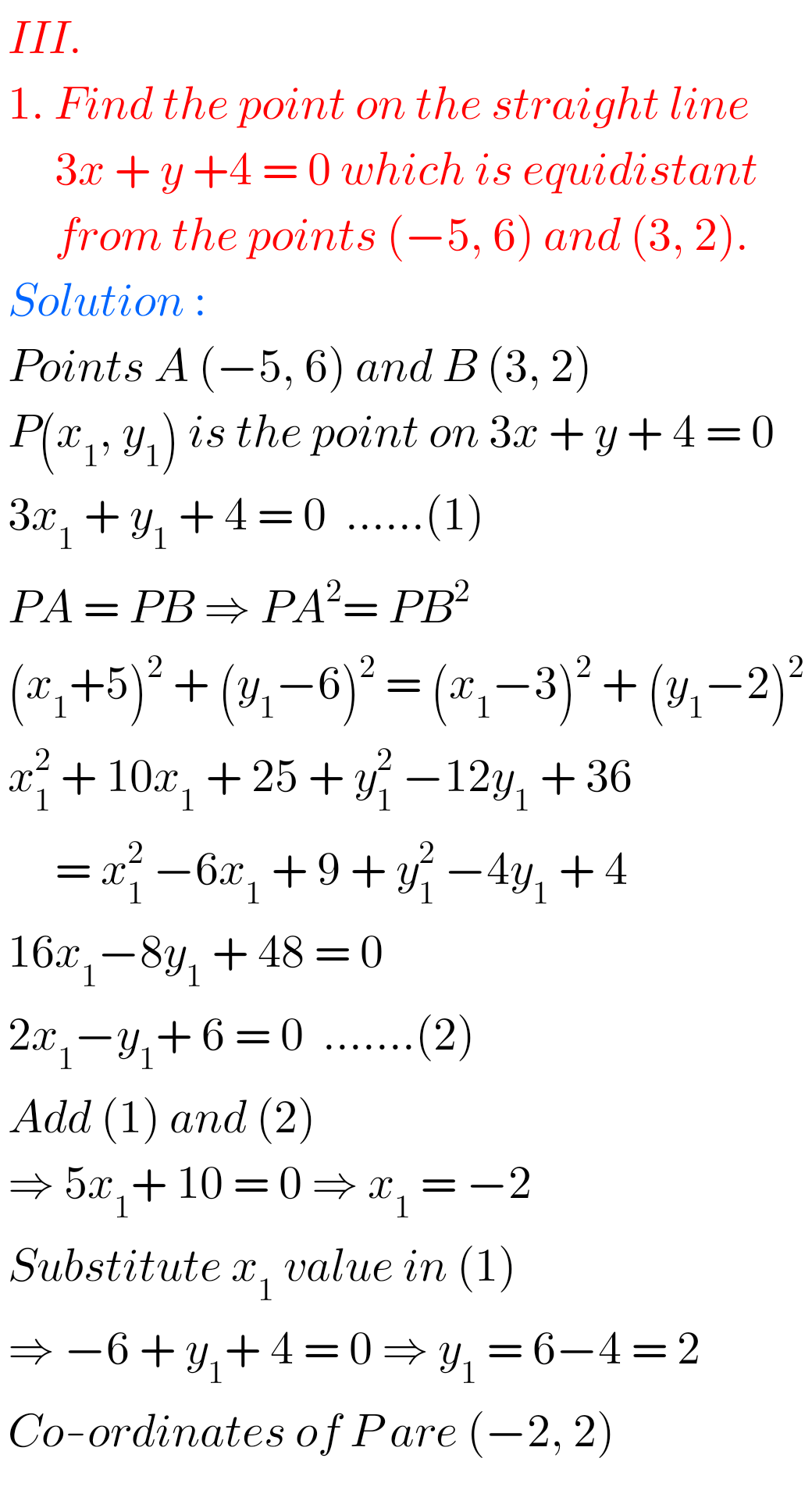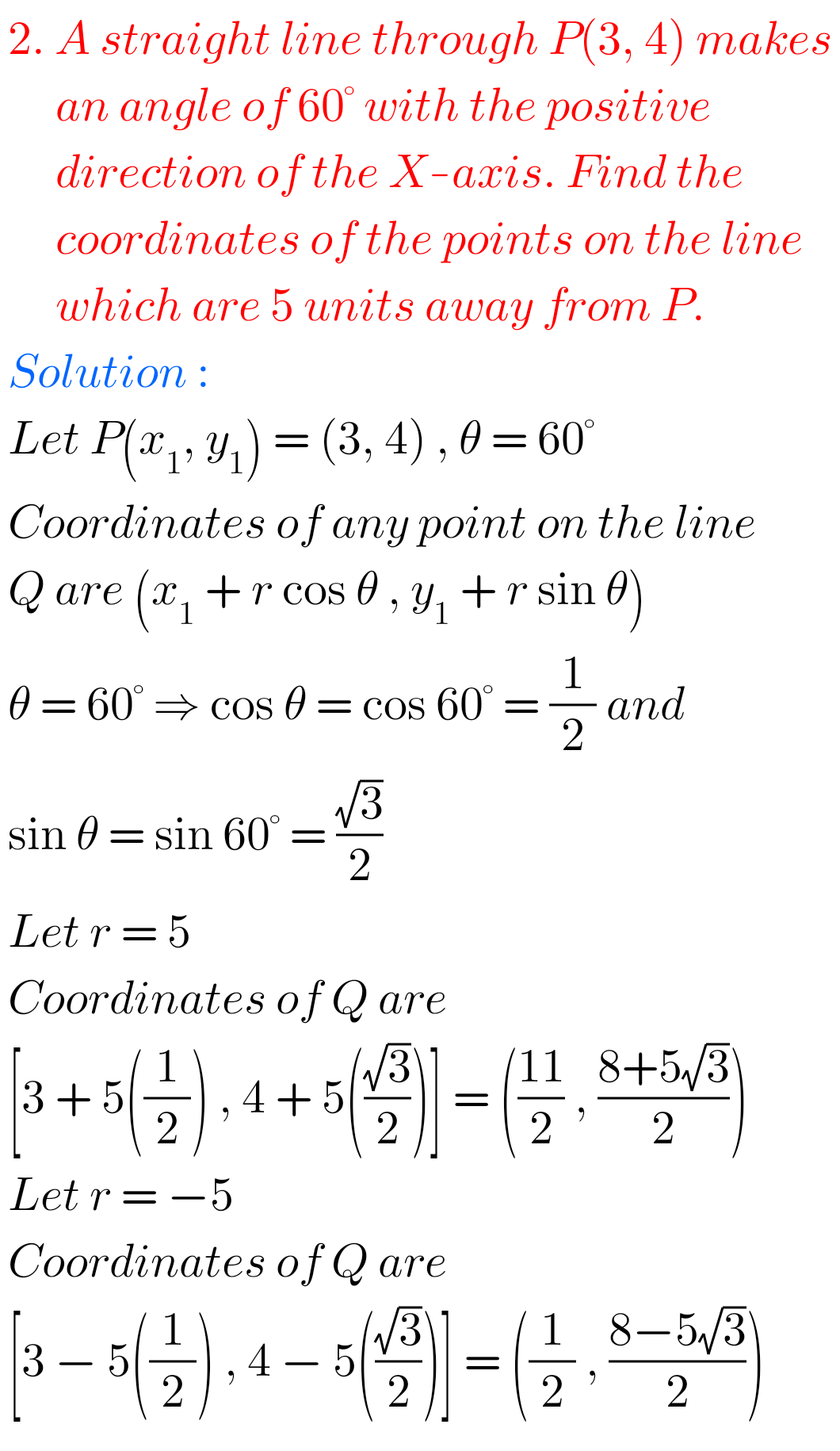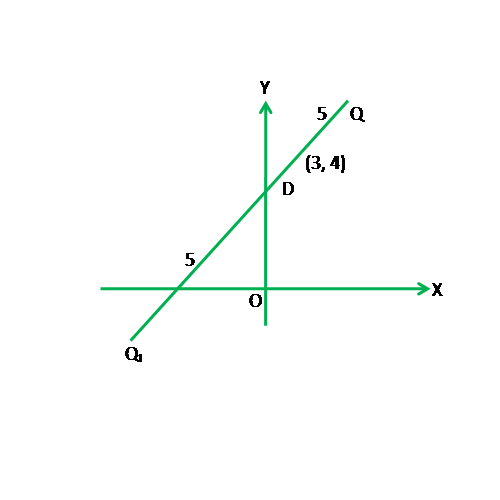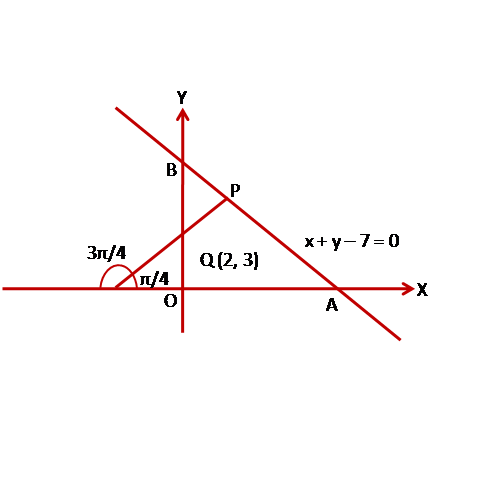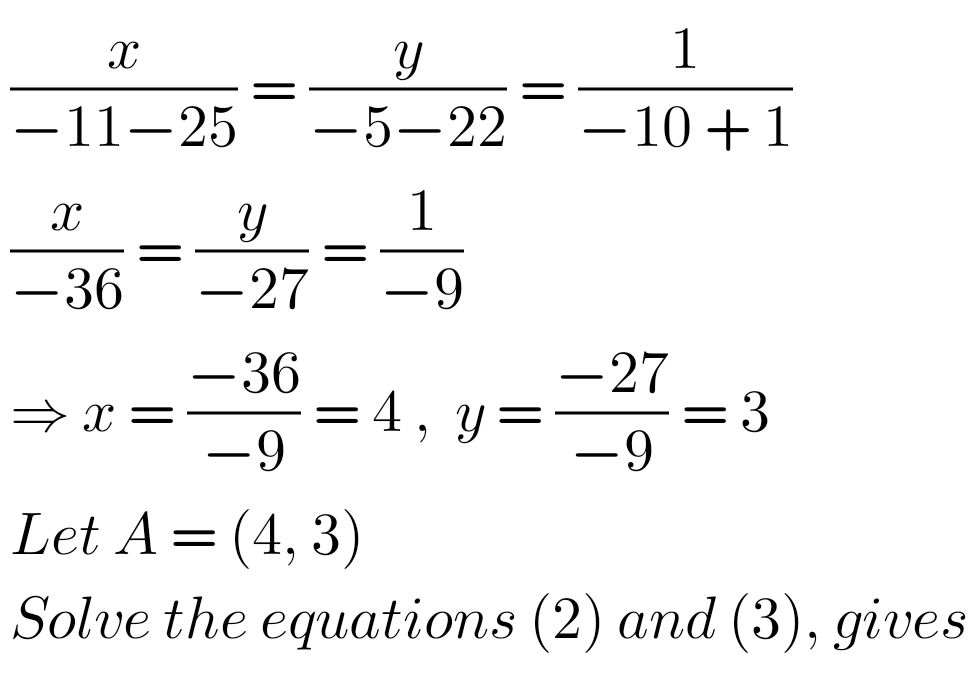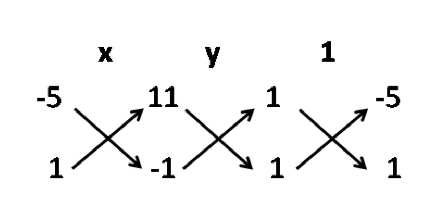Note : Observe the solutions and try them in your own method.

SSC Maths solutions for class 10

Inter Maths 1A textbook solutions

Ncert maths class 6 chapter 14 solutions

Nios maths 311 book 1 Sets 1.1 solutions